# Texas Go Math Grade 3 Lesson 18.8 Answer Key Metric Units for Mass

Refer to our Texas Go Math Grade 3 Answer Key Pdf to score good marks in the exams. Test yourself by practicing the problems from Texas Go Math Grade 3 Lesson 18.8 Answer Key Metric Units for Mass.

## Texas Go Math Grade 3 Lesson 18.8 Answer Key Metric Units for Mass

Unlock the Problem

Peter has a dollar bill in his pocket. Should Peter measure the mass of the dollar bill in grams or kilograms?

The gram (g) is the basic metric unit for measuring mass, or the amount of matter in an object. Mass can also be measured by using the metric unit kilogram (kg).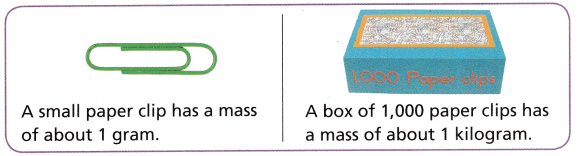Think: The mass of a dollar bill is closer to the mass of a small paper clip than it is to a box of 1,000 paper clips.
So, Peter should measure the mass of the dollar bill in ___________.
Grams

Explanation:
Peter should measure the mass of the dollar bill in grams.

Activity 1

Materials; pan balance, gram and kilogram masses

You can use a pan balance to measure mass.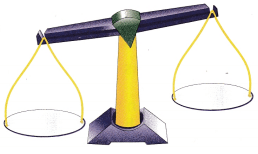Do 10 grams have the same mass as 1 kilogram?

• Place 10 gram masses on one side of the balance.
• Place a 1-kilogram mass on the other side of the balance.

Think: If it is balanced, then the objects have the same mass.
If it is not balanced, the objects do not have the same mass.

Complete the picture of the pan balance above by drawing in the masses.
The pan balance is __________.
So, 10 grams and 1 kilogram ___________ the same mass.
The pan balance is not balanced
10 grams and 1 kilogram do not have the same mass.

Explanation:Math Talk
Mathematical Processes

Which has a greater mass, 10 grams or 1 kilogram? Explain.
1 kilogram has a greater mass than 10 grams

Explanation:
The kilogram and gram are units to measure the mass. We can write kg for kilogram and g for gram.

Activity 2

Materials; pan balance, gram and kilogram masses,classroom objects

STEP 1:
Use the objects in the table. Decide if the object should be measured in grams or kilograms.

STEP 2:
Find the mass of each object to the nearest gram or kilogram. Place the object on one side of the balance. Place gram or kilogram masses on the other side until both sides are balanced.

STEP 3:
Add the measures of the gram or kilogram masses. This is the mass of the object. Record the mass in the table.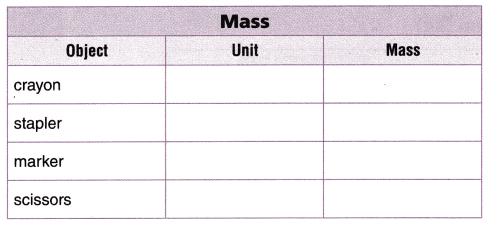Write the objects in order from greatest mass to least mass.
_________, __________, ____________, _____________
stapler, scissors, marker, crayon

Explanation:Share and Show

Question 1.
Five bananas have a mass of about ___________.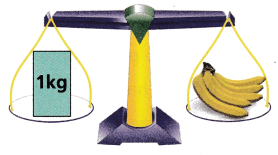Think: The pan balance is balanced, so the objects on both sides have the same mass.
1 kilogram

Explanation:
Five bananas have a mass of about 1 kilogram.

Choose the unit you would use to measure the mass. Write gram or kilogram.

Question 2.
strawberryGram

Question 3.
dog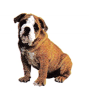Kilogram

Question 4.
sunglasses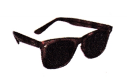Gram

Problem Solving

Question 5.
Put the sports balls shown at the right in order from greatest mass to least mass.
bowling ball, baseball, tennis ball, golf ball, table tennis ball.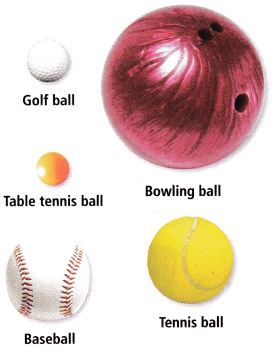Explanation:
The sports balls shown at the right in order from greatest mass to least mass are bowling balls, baseball, tennis balls, golf balls, and table tennis balls.

Go Math Metric Units for Mass Grade 3 Question 6.
H.O.T. Multi-Step One golf ball has a mass of about 46 grams. Mrs. Downs bought a box of 2 golf balls for her first round of golf and bought another box of 2 golf balls for the second round of golf. What is the total mass of the golf balls that Mrs. Downs bought? Represent your steps to solve the problem.
184 grams

Explanation:
From the given question we can show a box with 46 + 46 and a box with 46 + 46; add each box 92 + 92 = 184; hence we could also multiple 46 × 4 to get the answer.

Question 7.
H.O.T. Our dog, Dexter, weighs 72 kilograms. He is 8 times heavier than our cat. What does our cat weigh? Explain.
9 kilograms

Explanation:
I have divided 72 by 8 to get the answer is 9.

Question 8.
Use Diagrams Choose two objects that have different masses. Draw a balance with one of these objects on each side.

Question 9.
H.O.T. Sense or Nonsense?
Amber is buying produce at the grocery store. She says that a Fuji apple and a green bell pepper would have the same mass because they are the same size. Does her statement make sense? Explain.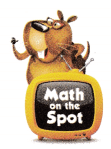No

Explanation:
Because the two objects are of the same size does not mean that they have the same mass. The mass of the apple may be greater because it is solid inside.

Fill in the bubble for the correct answer choice.

Question 10.
A turkey vulture weighs about as much as a laptop computer. Which is the best unit of measure to find the mass of a turkey vulture?
(A) liter
(B) kilogram
(C) ounce
(D) gram
Kilogram

Explanation:
The kilogram is the best unit of measure to find the mass of a turkey vulture.

Question 11.
Which item’s mass should be measured in grams?Option B

Explanation:
Option B item’s mass should be measured in grams.

Question 12.
Multi-Step The third-grade classes are making three salt maps on a thin piece of plywood. Together the three maps will have a total mass of 6 kilograms. If the salt mass is about half the mass of each map, which is the mass of salt needed for each map?
(A) 1 kilogram
(B) 5cups
(C) 3 liters
(D) 5grams
1 kilogram

Explanation:
The mass of salt needed for each map is 1 kilogram.

Texas Test Prep

Question 13.
Dan wants to find the mass of a large pumpkin. Which unit should he use?
(A) inch
(B) gram
(C) kilogram
(D) liter
Kilogram

Explanation:
The unit he should use is a kilogram.

### Texas Go Math Grade 3 Lesson 18.8 Homework and Practice Answer Key

Choose the unit you would use to measure the mass. Write gram or kilogram.

Question 1.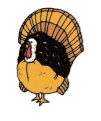Kilogram

Question 2.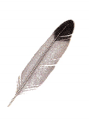gram

Question 3.Gram

Question 4.Kilogram

Question 5.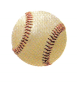Gram

Question 6.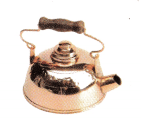Kilogram

Problem Solving

Question 7.
A bag of peanuts weighs about 36 grams. A bag of walnuts weighs about 42 grams. Yola buys 2 bags of each. How many grams of nuts does Yola have? Represent your steps to solve the problem.
156 grams

Explanation:
We can show a box with 36 + 36 and a box with 42 + 42; we can add each box 72 + 84 = 156. We can also add 36 + 42 = 78 and then multiply 2 × 78 = 156.

Question 8.
Look at the balance scale below. Draw an object on one side of the scale to make the scale balanced.Lesson Check

Question 9.
A juice box weighs about as much as a softball. Which is the best unit to use to measure the mass of a juice box?
(A) gram
(B) pound
(C) kilogram
(D) milliliter
Gram

Explanation:
The best unit to use to measure the mass of a juice box is the gram.

Question 1o.
Antonio wants to find the mass of his backpack filled with books. Which unit should he use?
(A) ounce
(B) gram
(C) kilogram
(D) quart
Kilogram

Explanation:
The unit used is the kilogram

Texas Go Math Grade 3 Metric Units of Mass Question 11.
Which item’s mass should be measured in kilograms?Option D

Explanation:
Option D item’s mass should be measured in kilogram.

Question 12.
Which item’s mass should be measured in grams?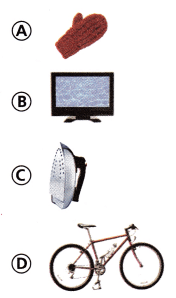Option A

Explanation:
Option A item’s mass should be measured in grams.

Question 13.
Multi-Step Sarah is making fruit salad for a picnic. She slices 3 kilograms of apples, 5 kilograms of peaches, and 2 kilograms of strawberries. She divides the fruit into 5 large bowls. About how many kilograms of fruit are in each bowl?
(A) 12 kilograms
(B) 2 kilograms
(C) 7 kilograms
(D) 10 kilograms
2 kilograms

Explanation:
3 kilograms of apples, 5 kilograms of peaches, and 2 kilograms of strawberries = 10 kg
Divides the fruit into 5 large bowls = 10÷5 = 2 kg

Question 14.
Multi-Step Desiree is baking cakes. She needs 9 grams of baking powder and 12 grams of baking soda. She divides the baking powder and baking soda equally among 3 pies. How many combined grams of baking powder and baking soda will each pie get?
(A) 21 grams
(B) 7 grams
(C) 24 grams
(D) 8 grams